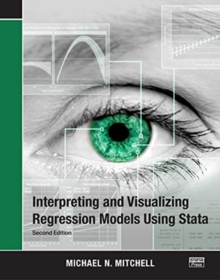Interpreting and Visualizing Regression Models Using Stata

76,90>>>>>NOTA BENE: QUESTO LIBRO È DISPONIBILE IN CIRCA 10 GIORNI LAVORATIVI DALLA DATA DELL'ORDINE<<<<<

COD: 9781597183215 Categoria:

Description

Interpreting and Visualizing Regression Models Using Stata, Second Edition provides clear and simple examples illustrating how to interpret and visualize a wide variety of regression models. Including over 200 figures, the book illustrates linear models with continuous predictors (modeled linearly, using polynomials, and piecewise), interactions of continuous predictors, categorical predictors, interactions of categorical predictors, and interactions of continuous and categorical predictors. The book also illustrates how to interpret and visualize results from multilevel models, models where time is a continuous predictor, models with time as a categorical predictor, nonlinear models (such as logistic or ordinal logistic regression), and models involving complex survey data.

The examples illustrate the use of the margins, marginsplot, contrast, and pwcompare commands. This new edition reflects new and enhanced features added to Stata, most importantly the ability to label statistical output using value labels associated with factor variables. As a result, output regarding marital status is labeled using intuitive labels like Married and Unmarried instead of using numeric values such as 1 and 2.

All the statistical output in this new edition capitalizes on this new feature, emphasizing the interpretation of results based on variables labeled using intuitive value labels. Additionally, this second edition illustrates other new features, such as using transparency in graphics to more clearly visualize overlapping confidence intervals and using small sample-size estimation with mixed models. If you ever find yourself wishing for simple and straightforward advice about how to interpret and visualize regression models using Stata, this book is for you.

Dettagli

Anno 2021 610 Brossura con copertina morbida Inglese 2 New edition MITCHELL MICHAEL N. STATA PRESS